# DAV Class 3 Maths Chapter 5 Worksheet 10 Solutions

The DAV Class 3 Maths Book Solutions and DAV Class 3 Maths Chapter 5 Worksheet 10 Solutions of Division offer comprehensive answers to textbook questions.

## DAV Class 3 Maths Ch 5 WS 10 Solutions

Solve the following:

Question 1.Solution:Quotient = 53
Remainder = 5

Question 2.Solution:Quotient = 69
Remainder = 3

Question 3.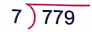Solution:Quotient = 111
Remainder = 2Question 4.Solution: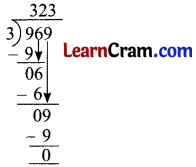Quotient = 323
Remainder = 0

Question 5.Solution: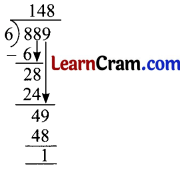Quotient = 148
Remainder = 1

Question 6.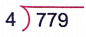Solution:Quotient = 194
Remainder = 0Question 7.Solution: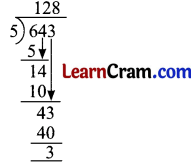Quotient = 128
Remainder = 3

Question 8.Solution: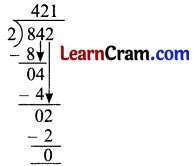Quotient = 421
Remainder = 0

Question 9.Solution: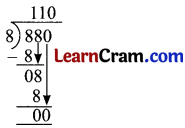Quotient = 110
Remainder = 0### DAV Class 3 Maths Chapter 5 Worksheet 10 Notes

Division by 3-Digit Numbers

576 ÷ 5

576 = 5 hundreds + 7 tens + 6 ones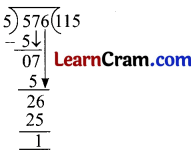Quotient = 115,
Remainder = 1